# Visual Prostheses¶

Objects in the implants module are organized into the following categories:

## Prosthesis systems¶

pulse2percept provides the following prosthesis systems (aka ‘retinal implants’, ‘bionic eyes’):

 Implant Location Num Electrodes Manufacturer ArgusI epiretinal 16 Second Sight Medical Products Inc ArgusII epiretinal 60 Second Sight Medical Products Inc IMIE epiretinal 256 IntelliMicro Medical Co., Ltd AlphaIMS subretinal 1500 Retina Implant AG AlphaAMS subretinal 1600 Retina Implant AG PRIMA subretinal 378 Pixium Vision SA PRIMA75 subretinal 142 Pixium Vision SA PRIMA55 subretinal 273(?) Pixium Vision SA PRIMA40 subretinal 532(?) Pixium Vision SA BVT24 suprachoroidal 24 Bionic Vision Technologies BVT44 suprachoroidal 44 Bionic Vision Technologies

Stimuli can be assigned to the various electrodes in the electrode array, who will deliver them to the retina (see Electrical Stimuli). A mathematical model is then used to compute the neural stimulus response and predict the resulting visual percept (see Computational Models).

### Understanding the coordinate system¶

The easiest way to understand the coordinate system is to look at the organization of the optic fiber layer:

In : from pulse2percept.models import AxonMapModel

In : AxonMapModel(eye='RE').plot()
Out: <AxesSubplot:xlabel='x (microns)', ylabel='y (microns)'>


Here you can see that:

• the coordinate system is centered on the fovea
• in a right eye, positive $$x$$ values correspond to the nasal retina
• in a right eye, positive $$y$$ values correspond to the superior retina

Positive $$z$$ values move an electrode away from the retina into the vitreous humor ($$z$$ is sometimes called electrode-retina distance). Analogously, negative $$z$$ values move an electrode through the different retinal layers towards the outer retina.

### Understanding the ProsthesisSystem class¶

The ProsthesisSystem base class provides a template for all prosthesis systems. It is comprised of:

### Accessing electrodes¶

You can access individual electrodes in a prosthesis system either by integer index or by electrode name. For example, the first electrode in AlphaAMS can be accessed as follows:

In : from pulse2percept.implants import AlphaAMS

In : implant = AlphaAMS()

# Access by index:
In : implant
Out:
DiskElectrode(activated=True, name='A1', r=15.0, x=-1365.0,
y=-1365.0, z=0.0)

# Access by name:
In : implant['A1']
Out:
DiskElectrode(activated=True, name='A1', r=15.0, x=-1365.0,
y=-1365.0, z=0.0)


The simplest way to iterate over all electrodes is to pretend that the prosthesis system is a Python dictionary:

In : from pulse2percept.implants import ArgusI

In : for name, electrode in ArgusI().electrodes.items():
...:     print(name, electrode)
...:
A1 DiskElectrode(activated=True, name='A1', r=125.0,
x=-1200.0, y=-1200.0, z=0.0)
B1 DiskElectrode(activated=True, name='B1', r=250.0, x=-400.0,
y=-1200.0, z=0.0)
C1 DiskElectrode(activated=True, name='C1', r=125.0, x=400.0,
y=-1200.0, z=0.0)
D1 DiskElectrode(activated=True, name='D1', r=250.0, x=1200.0,
y=-1200.0, z=0.0)
A2 DiskElectrode(activated=True, name='A2', r=250.0,
x=-1200.0, y=-400.0, z=0.0)
B2 DiskElectrode(activated=True, name='B2', r=125.0, x=-400.0,
y=-400.0, z=0.0)
C2 DiskElectrode(activated=True, name='C2', r=250.0, x=400.0,
y=-400.0, z=0.0)
D2 DiskElectrode(activated=True, name='D2', r=125.0, x=1200.0,
y=-400.0, z=0.0)
A3 DiskElectrode(activated=True, name='A3', r=125.0,
x=-1200.0, y=400.0, z=0.0)
B3 DiskElectrode(activated=True, name='B3', r=250.0, x=-400.0,
y=400.0, z=0.0)
C3 DiskElectrode(activated=True, name='C3', r=125.0, x=400.0,
y=400.0, z=0.0)
D3 DiskElectrode(activated=True, name='D3', r=250.0, x=1200.0,
y=400.0, z=0.0)
A4 DiskElectrode(activated=True, name='A4', r=250.0,
x=-1200.0, y=1200.0, z=0.0)
B4 DiskElectrode(activated=True, name='B4', r=125.0, x=-400.0,
y=1200.0, z=0.0)
C4 DiskElectrode(activated=True, name='C4', r=250.0, x=400.0,
y=1200.0, z=0.0)
D4 DiskElectrode(activated=True, name='D4', r=125.0, x=1200.0,
y=1200.0, z=0.0)


### Creating your own prosthesis system¶

You can quickly create a prosthesis system from an ElectrodeArray (or even a single Electrode) by wrapping it in a ProsthesisSystem container:

In : from pulse2percept.implants import ElectrodeGrid, ProsthesisSystem

In : ProsthesisSystem(earray=ElectrodeGrid((10, 10), 200))
Out:
ProsthesisSystem(earray=ElectrodeGrid, eye='RE',
preprocess=False, safe_mode=False,
stim=None)


To create a more advanced prosthesis system, you will need to subclass the base class:

import numpy as np
from pulse2percept.implants import ElectrodeGrid, ProsthesisSystem

class MyFovealElectrodeGrid(ProsthesisSystem):
"""An ElectrodeGrid implant centered over the fovea"""

def __init__(self, stim=None, eye='RE'):
self.earray = ElectrodeGrid((3, 3), x=0, y=0, z=0, rot=0,
r=100, spacing=500,
names=('A', '1'))
self.stim = stim
self.eye = eye

def check_stim(self, stim):
"""Make sure the stimulus is charge-balanced"""
if stim.time is not None:
for s in stim:
assert np.isclose(np.sum(s), 0)


#### Examples using ProsthesisSystem¶Simulating Argus II

Simulating Argus II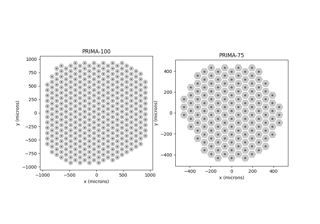Retinal implant gallery

Retinal implant gallery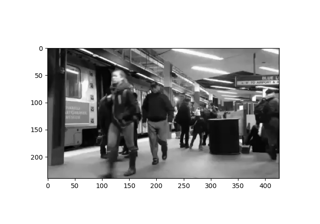Generating a stimulus from a video

Generating a stimulus from a video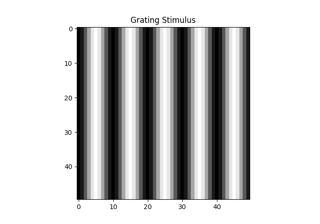Generating a drifting sinusoidal grating or drifting bar stimulus

Generating a drifting sinusoidal grating or drifting bar stimulus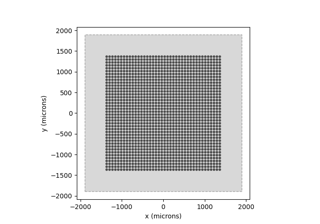Generating a stimulus from an image

Generating a stimulus from an imageThompson et al. (2003): Circular phosphenes

Thompson et al. (2003): Circular phosphenes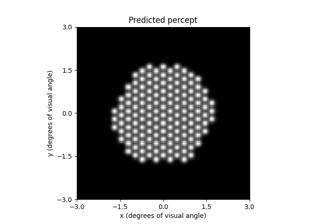Beyeler et al. (2019): Focal percepts with the scoreboard model

Beyeler et al. (2019): Focal percepts with the scoreboard model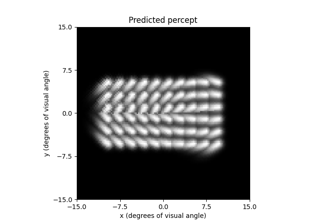Beyeler et al. (2019): Axonal streaks with the axon map model

Beyeler et al. (2019): Axonal streaks with the axon map modelRetinotopy: Predicting the perceptual effects of different visual field maps

Retinotopy: Predicting the perceptual effects of different visual field maps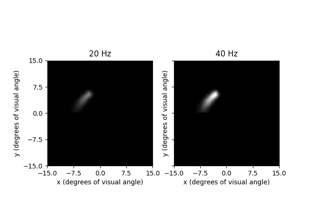Granley et al. (2021): Effects of Biphasic Pulse Parameters with the BiphasicAxonMapModel

Granley et al. (2021): Effects of Biphasic Pulse Parameters with the BiphasicAxonMapModel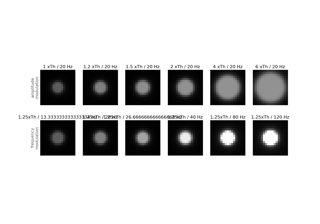Nanduri et al. (2012): Frequency vs. amplitude modulation

Nanduri et al. (2012): Frequency vs. amplitude modulation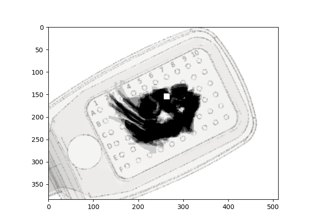Phosphene drawings from Beyeler et al. (2019)

Phosphene drawings from Beyeler et al. (2019)

## Electrode arrays¶

Electrode arrays are collections of Electrode objects whose behavior is dictated by the ElectrodeArray base class.

### Understanding the ElectrodeArray class¶

The ElectrodeArray base provides:

• electrodes: an ordered dictionary of electrode objects (meaning it will remember the order in which electrodes were added),
• n_electrodes: a property returning the number of electrodes in the array.
• add_electrode: a method to add a single electrode to the collection,
• add_electrodes: a method to add a multiple electrodes to the collection at once,
• a way to access a single electrode either by index or by name,
• a way to iterate over all electrodes in the array.

### Accessing electrodes¶

You can access individual electrodes in an electrode array either by integer index or by electrode name. The syntax is exactly the same as for the prosthesis system.

### Creating your own electrode array¶

You can create your own electrode array by starting with an empty ElectrodeArray, and adding the desired electrodes one by one:

In : from pulse2percept.implants import DiskElectrode, ElectrodeArray

In : earray = ElectrodeArray([])

In : earray.add_electrode(0, DiskElectrode(0, 0, 0, 50))

In : earray.add_electrode(1, DiskElectrode(100, 100, 0, 150))

In : earray
Out: ElectrodeArray(electrodes=OrderedDict, n_electrodes=2)


To create a more advanced electrode array, you will need to subclass the base class. In the constructor, make sure to initialize self.electrodes with an ordered dictionary (OrderedDict):

from collections import OrderedDict
from pulse2percept.implants import ElectrodeArray

class MyElectrodeArray(ElectrodeArray):
"""Array with a single disk electrode"""

def __init__(self, name):
self.electrodes = OrderedDict()


#### Examples using ElectrodeArray¶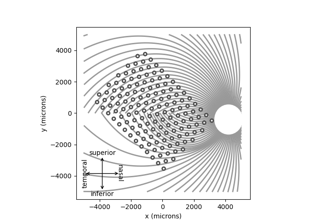Creating a grid of electrodes

Creating a grid of electrodes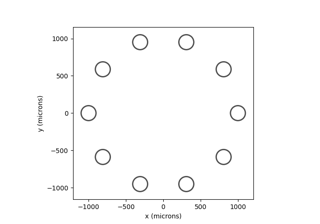Nanduri et al. (2012): Frequency vs. amplitude modulation

Nanduri et al. (2012): Frequency vs. amplitude modulation

## Electrodes¶

Electrodes are objects whose behavior is dictated by the Electrode base class. They are located at a particular 3D location and provide a method to calculate the electric potential at arbitrary 3D locations.

### Understanding the Electrode class¶

The base class provides:

• the 3D coordinates of the center of the electrode.

In addition, a custom electrode object must implement:

• a method called electric_potential that returns the electric potential at a point (x, y, z).

To create a new electrode type, you will need to subclass the base class. Make sure to specify an electric_potential method for your class:

from pulse2percept.implants import Electrode

class MyElectrode(Electrode):
"""Named electrode with electric potential 0 everywhere"""

def __init__(self, x, y, z, name):
# Note: If you don't plan on adding any new variables, you can
# omit the constructor entirely. In that case, your object will
# inherit the constructor of the base class.
self.x = x
self.y = y
self.z = z
self.name = name

def electric_potential(self, x, y, z):
return 0.0


#### Examples using Electrode¶Creating a grid of electrodes

Creating a grid of electrodes